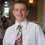# Exponential Integral and Euler-Mascheroni Constant

$\large \lim_{x\to 0^{+}} \left( \text{Ei}(-x) - \ln(x)\right) = \gamma$

EDIT: I figured out a solution; see my comment below.

To clarify:

• $\text{Ei}(y) = \displaystyle\int_{-y}^{\infty} \dfrac{-e^{-t}}{t} dt = \displaystyle\int_{-\infty}^{y} \dfrac{e^t}{t}dt$ denotes the exponential integral;

• $\gamma$ denotes the Euler-Mascheroni constant, $\gamma \approx 0.5772$.

• Notation: $H_n$ denotes the $n^\text{th}$ harmonic number, $H_n = 1 + \dfrac12 + \dfrac13 + \cdots + \dfrac1n$.

I came across this limit when I was trying to derive the derivative of the factorial function: $\dfrac{d(n!)}{dn} = n!(H_n - \gamma)$ for all whole numbers $n$. Let's denote the derivative as $D(n)$ We know that $(n+1)! = (n+1)n!$. Therefore, differentiating gives us:

$D(n+1) = (n+1)D(n) + n!$

$\dfrac{D(n+1)}{(n+1)!} = \dfrac{(n+1)D(n)}{(n+1)!} + \dfrac{n!}{(n+1)!} = \dfrac{D(n)}{n!} + \dfrac{1}{n+1}$

It follows that $\dfrac{D(n)}{n!} = H_n + a$ for some constant $a$. What I can't figure out is how to prove that $a = -\gamma$. I tried to find the value of $D(0)$:

$D(n) = \dfrac{d}{dn}\left(\int_{0}^{\infty} x^n e^{-x} dx \right) = \int_{0}^{\infty} \dfrac{d(x^n e^{-x})}{dn} dx$

$D(n) = \int_{0}^{\infty} x^n \ln(x) e^{-x} dx$

Therefore, $D(0) = \int_{0}^{\infty} \ln(x) e^{-x} dx$ Using integration by parts, this becomes:

$D(0) = -e^{-x} \ln(x) \rvert_{0}^{\infty} - \int_{0}^{\infty} \dfrac{-e^{-x}}{x}dx$

$=\lim_{y\to 0^{+}} \left[ -e^{-x} \ln(x) \rvert_{y}^{\infty} - \int_{y}^{\infty} \dfrac{-e^{-x}}{x}dx \right]$

$D(0) = \lim_{y\to 0^{+}} (e^{-y} \ln(y) - \text{Ei}(-y))$

We can simplify this a little by observing the following: $\lim_{y\to 0^{+}} (e^{-y} - 1) \ln(y) = \lim_{y\to 0^{+}} \dfrac{e^{-y} - 1}{-y} \lim_{y\to 0^{+}} (-y\ln(y)) = 1 * 0 = 0$

Hence, the equation for $D(0)$ can simplify to:

$D(0) = \lim_{y\to 0^{+}} (\ln(y) - \text{Ei}(-y))$

Now this is supposed to equal $-\gamma$, but I cannot figure out how to prove it, and can't seem to find the proof anywhere either.Note by Ariel Gershon
5 years, 2 months ago

This discussion board is a place to discuss our Daily Challenges and the math and science related to those challenges. Explanations are more than just a solution — they should explain the steps and thinking strategies that you used to obtain the solution. Comments should further the discussion of math and science.

When posting on Brilliant:

• Use the emojis to react to an explanation, whether you're congratulating a job well done , or just really confused .
• Ask specific questions about the challenge or the steps in somebody's explanation. Well-posed questions can add a lot to the discussion, but posting "I don't understand!" doesn't help anyone.
• Try to contribute something new to the discussion, whether it is an extension, generalization or other idea related to the challenge.

MarkdownAppears as
*italics* or _italics_ italics
**bold** or __bold__ bold
- bulleted- list
• bulleted
• list
1. numbered2. list
1. numbered
2. list
Note: you must add a full line of space before and after lists for them to show up correctly
paragraph 1paragraph 2

paragraph 1

paragraph 2

[example link](https://brilliant.org)example link
> This is a quote
This is a quote
    # I indented these lines
# 4 spaces, and now they show
# up as a code block.

print "hello world"
# I indented these lines
# 4 spaces, and now they show
# up as a code block.

print "hello world"
MathAppears as
Remember to wrap math in $$ ... $$ or $ ... $ to ensure proper formatting.
2 \times 3 $2 \times 3$
2^{34} $2^{34}$
a_{i-1} $a_{i-1}$
\frac{2}{3} $\frac{2}{3}$
\sqrt{2} $\sqrt{2}$
\sum_{i=1}^3 $\sum_{i=1}^3$
\sin \theta $\sin \theta$
\boxed{123} $\boxed{123}$

Sort by:

By definition, $\displaystyle \text{Ei}(x) = -\int_{-x}^\infty \dfrac{e^{-t}}t \, dt$ and $\displaystyle e^{-t} = \sum_{k=0}^\infty \dfrac{(-1)^{k+1} t^k }{k!}$. Integrating the Taylor series of $\dfrac {e^{-t}}t$, we get

$\text{Ei}(x) = \gamma + \ln| x| + \sum_{k=1}^\infty \dfrac{x^k}{k \cdot k!} \qquad x\ne 0 .$

Take the limit and the result follows.

- 5 years, 2 months ago

Here's the thing though - how do you know the first term is $\gamma$ ??

- 5 years, 2 months ago

Hey Ariel, I see that you like to solve difficult calculus questions. We got a group on another social platform (online forum) that discusses all kinds of difficult calculus questions as well. Plus, most of the active members on Brilliant are on this online forum as well (even the staffs!). We discuss about everything related to math and science. Plus, it's free! Would you like to join us?

If yes, register here

See you!

- 5 years, 2 months ago

Just joined! Thanks! :)

- 5 years, 2 months ago

- 5 years, 2 months ago

I've figured out the solution after a while. Here I will use the generalized harmonic number: $H_x = \sum_{k=1}^{\infty} \left(\dfrac{1}{k} - \dfrac{1}{x+k}\right)$ Now let's start from the point where $\dfrac{D(n)}{n!} = H_n + a$. Let's integrate this on both sides from $0$ to $1$: $\int_{0}^{1} \dfrac{D(n)}{n!} dn = \int_{0}^{1} (H_n + a) dn$ $\ln(n!) \large{\rvert_0^1} \normalsize{ = a + \int_{0}^{1} \sum_{k=1}^{\infty} \left(\dfrac{1}{k} - \dfrac{1}{n+k}\right) dn}$ $0 = a + \sum_{k=1}^{\infty} \int_{0}^{1} \left(\dfrac{1}{k} - \dfrac{1}{n+k}\right) dn$ $-a = \sum_{k=1}^{\infty} \left(\dfrac{n}{k} - \ln(n+k)\right) \large{\rvert_0^1}$ $-a = \sum_{k=1}^{\infty} \left(\dfrac{1}{k} - \ln(1+k) + \ln(k)\right)$ $a = -\lim_{m \to \infty} \left(\sum_{k = 1}^{m} \dfrac{1}{k} - \ln(1+m)\right)$ $a = -\gamma$

- 5 years, 2 months ago